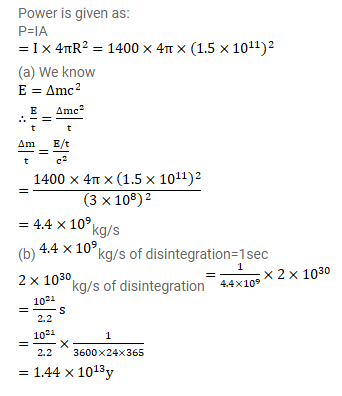# The energy from the sun reaches just outside the earth's atmosphere

Question:

The energy from the sun reaches just outside the earth's atmosphere at a rate of $1400 \mathrm{w} / \mathrm{m}^{2}$. The distance between the sun and the earth is $1.5 \times 10^{11} \mathrm{~m}$. (a) Calculate the rate at which the sun is losing its mass. (b) How long will the sun last assuming a constant decay at this rate? The present mass of the sun is $2 \times 10^{30} \mathrm{~kg}$.

Solution: